# ML | Mean-Shift Clustering

Meanshift is falling under the category of a clustering algorithm in contrast of Unsupervised learning that assigns the data points to the clusters iteratively by shifting points towards the mode (mode is the highest density of data points in the region, in the context of the Meanshift). As such, it is also known as the Mode-seeking algorithm. Mean-shift algorithm has applications in the field of image processing and computer vision.

Given a set of data points, the algorithm iteratively assigns each data point towards the closest cluster centroid and direction to the closest cluster centroid is determined by where most of the points nearby are at. So each iteration each data point will move closer to where the most points are at, which is or will lead to the cluster center. When the algorithm stops, each point is assigned to a cluster.

Unlike the popular K-Means cluster algorithm, mean-shift does not require specifying the number of clusters in advance. The number of clusters is determined by the algorithm with respect to the data.

Note: The downside to Mean Shift is that it is computationally expensive O(n²).

Mean-shift clustering is a non-parametric, density-based clustering algorithm that can be used to identify clusters in a dataset. It is particularly useful for datasets where the clusters have arbitrary shapes and are not well-separated by linear boundaries.

The basic idea behind mean-shift clustering is to shift each data point towards the mode (i.e., the highest density) of the distribution of points within a certain radius. The algorithm iteratively performs these shifts until the points converge to a local maximum of the density function. These local maxima represent the clusters in the data.

The process of mean-shift clustering algorithm can be summarized as follows:

Initialize the data points as cluster centroids.
Repeat the following steps until convergence or a maximum number of iterations is reached:
For each data point, calculate the mean of all points within a certain radius (i.e., the “kernel”) centered at the data point.
Shift the data point to the mean.
Identify the cluster centroids as the points that have not moved after convergence.
Return the final cluster centroids and the assignments of data points to clusters.
One of the main advantages of mean-shift clustering is that it does not require the number of clusters to be specified beforehand. It also does not make any assumptions about the distribution of the data, and can handle arbitrary shapes and sizes of clusters. However, it can be sensitive to the choice of kernel and the radius of the kernel.

Mean-Shift clustering can be applied to various types of data, including image and video processing, object tracking and bioinformatics.

### Kernel Density Estimation –

The first step when applying mean shift clustering algorithms is representing your data in a mathematical manner this means representing your data as points such as the set below.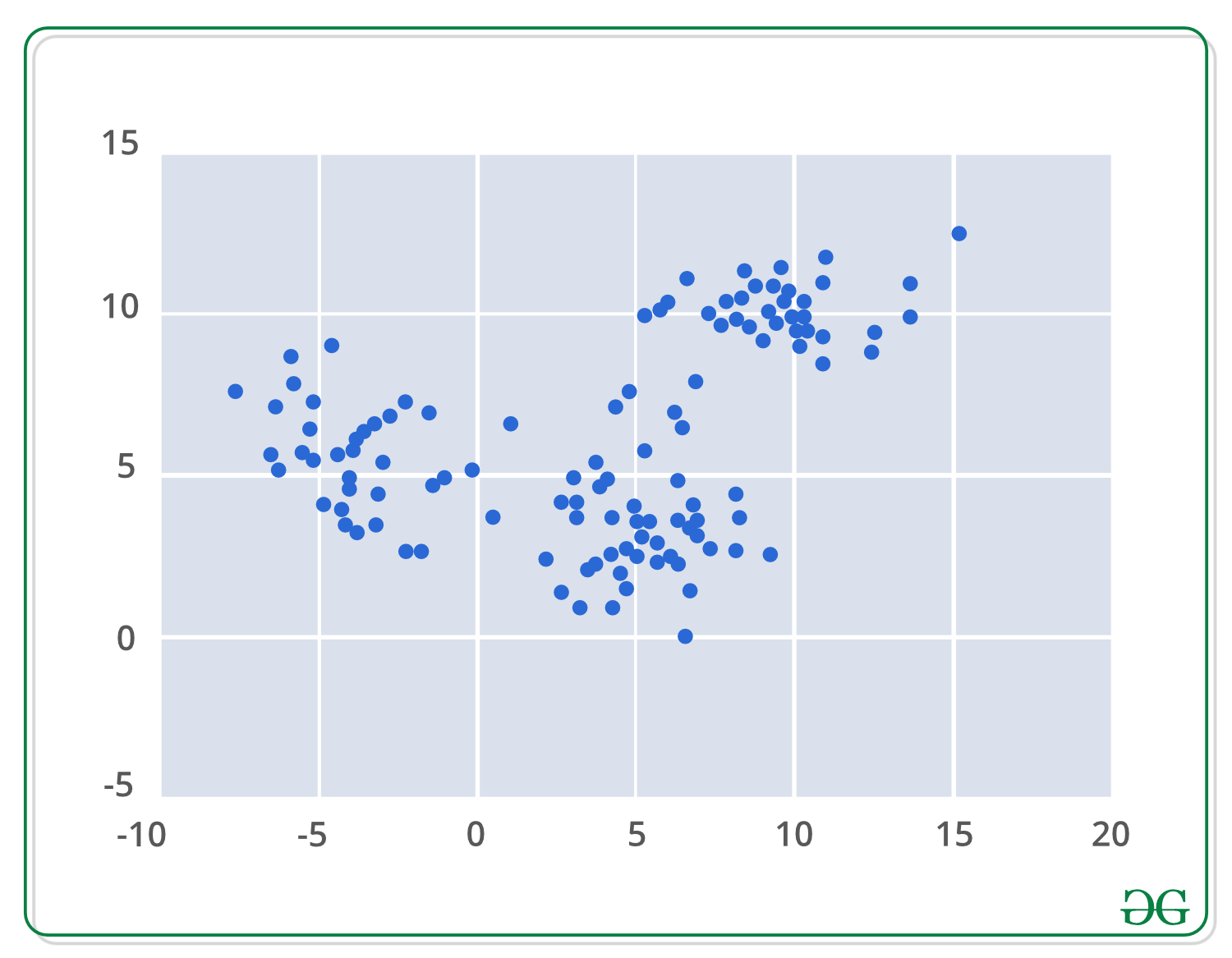Mean-shift builds upon the concept of kernel density estimation, in short KDE. Imagine that the above data was sampled from a probability distribution. KDE is a method to estimate the underlying distribution also called the probability density function for a set of data. It works by placing a kernel on each point in the data set. A kernel is a fancy mathematical word for a weighting function generally used in convolution. There are many different types of kernels, but the most popular one is the Gaussian kernel. Adding up all of the individual kernels generates a probability surface example density function. Depending on the kernel bandwidth parameter used, the resultant density function will vary. Below is the KDE surface for our points above using a Gaussian kernel with a kernel bandwidth of 2.

Surface plot: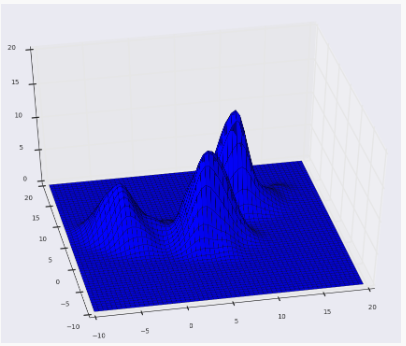Contour plot: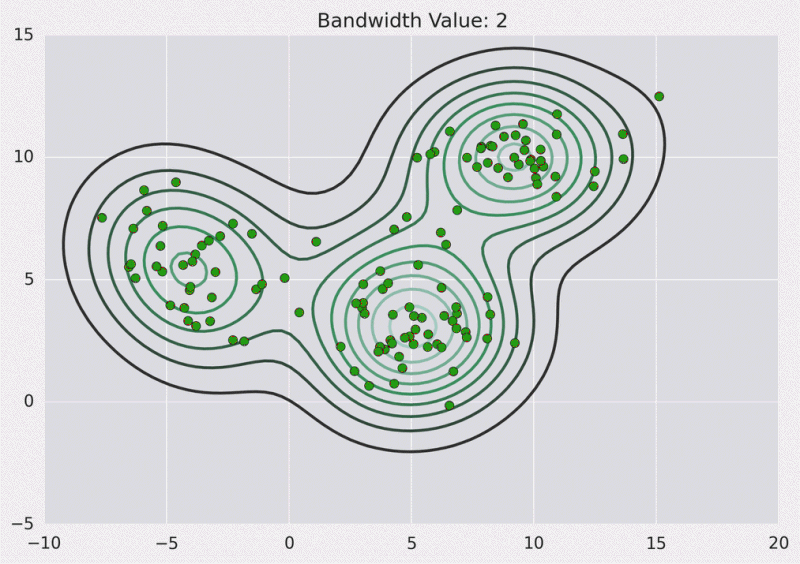Below is the Python implementation :

## Python3

 `import` `numpy as np` `import` `pandas as pd` `from` `sklearn.cluster ``import` `MeanShift` `from` `sklearn.datasets.samples_generator ``import` `make_blobs` `from` `matplotlib ``import` `pyplot as plt` `from` `mpl_toolkits.mplot3d ``import` `Axes3D` ` `  `# We will be using the make_blobs method` `# in order to generate our own data.`   `clusters ``=` `[[``2``, ``2``, ``2``], [``7``, ``7``, ``7``], [``5``, ``13``, ``13``]]`   `X, _ ``=` `make_blobs(n_samples ``=` `150``, centers ``=` `clusters,` `                                   ``cluster_std ``=` `0.60``)` ` `  `# After training the model, We store the` `# coordinates for the cluster centers` `ms ``=` `MeanShift()` `ms.fit(X)` `cluster_centers ``=` `ms.cluster_centers_` ` `  `# Finally We plot the data points` `# and centroids in a 3D graph.` `fig ``=` `plt.figure()`   `ax ``=` `fig.add_subplot(``111``, projection ``=``'3d'``)`   `ax.scatter(X[:, ``0``], X[:, ``1``], X[:, ``2``], marker ``=``'o'``)`   `ax.scatter(cluster_centers[:, ``0``], cluster_centers[:, ``1``],` `           ``cluster_centers[:, ``2``], marker ``=``'x'``, color ``=``'red'``,` `           ``s ``=` `300``, linewidth ``=` `5``, zorder ``=` `10``)`   `plt.show()`

Try Code here Output: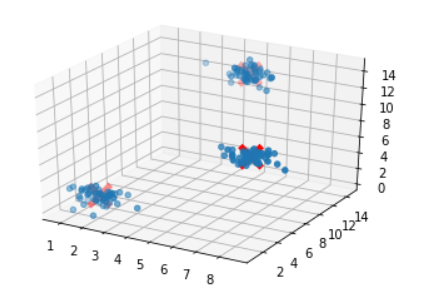To illustrate, suppose we are given a data set {ui} of points in d-dimensional space, sampled from some larger population, and that we have chosen a kernel K having bandwidth parameter h. Together, these data and kernel function returns the following kernel density estimator for the full population’s density function.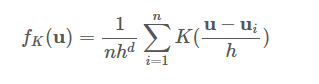The kernel function here is required to satisfy the following two conditions: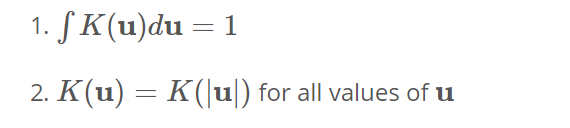-> The first requirement is needed to ensure that our estimate is normalized. -> The second is associated with the symmetry of our space.

Two popular kernel functions that satisfy these conditions are given by-Below we plot an example in one dimension using the Gaussian kernel to estimate the density of some population along the x-axis. We can see that each sample point adds a small Gaussian to our estimate, centered about it and equations above may look a bit intimidating, but the graphic here should clarify that the concept is pretty straightforward.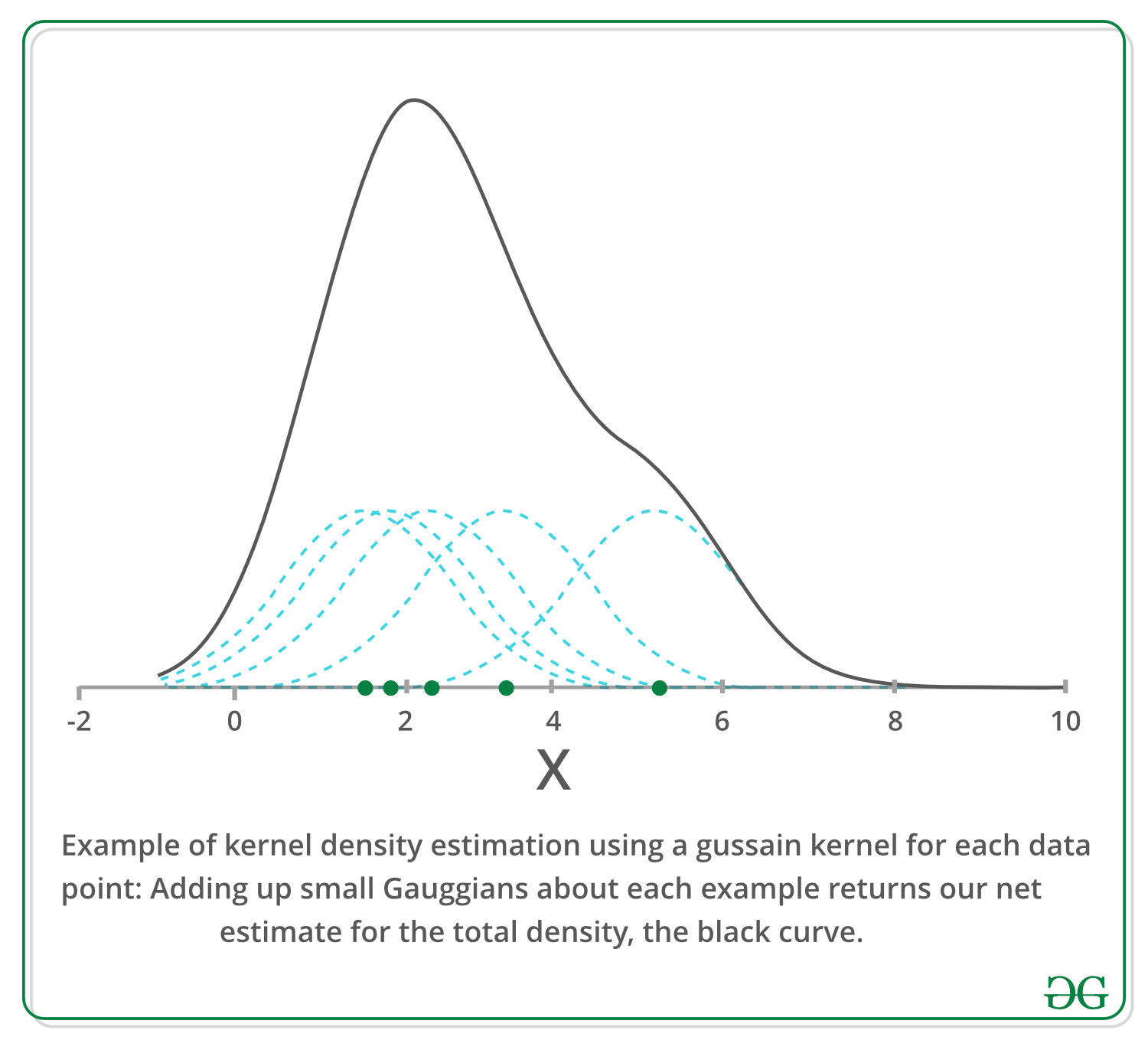Iterative Mode Search –

```1. Initialize random seed and window W.
2. Calculate the center of gravity (mean) of W.
3. Shift the search window to the mean.
4. Repeat Step 2 until convergence.```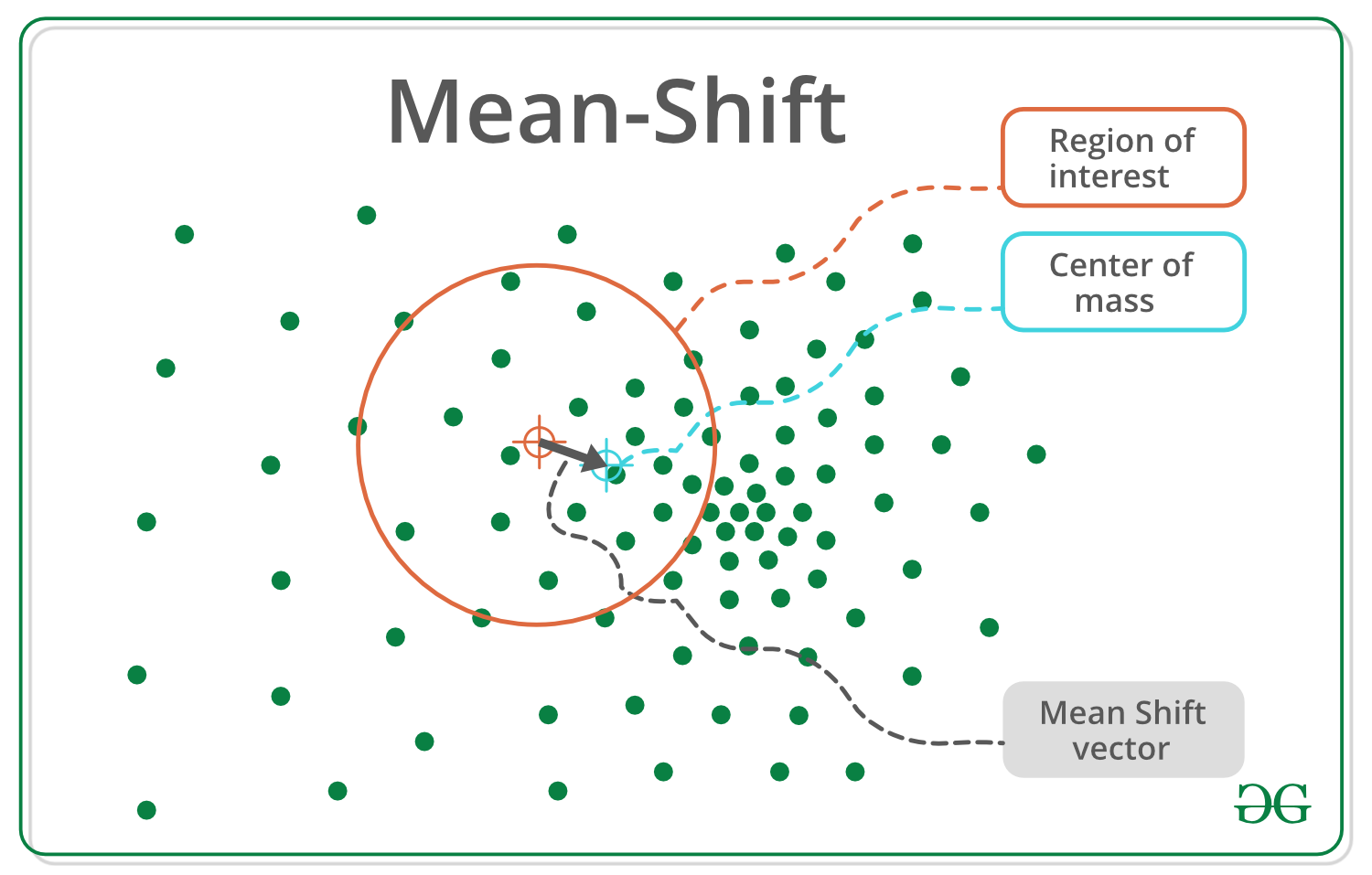General algorithm outline –

## Python3

 `for` `p ``in` `copied_points:` `    ``while` `not` `at_kde_peak:` `        ``p ``=` `shift(p, original_points)`

Shift function looks like this –

## Python3

 `def` `shift(p, original_points):` `    ``shift_x ``=` `float``(``0``)` `    ``shift_y ``=` `float``(``0``)` `    ``scale_factor ``=` `float``(``0``)`   `    ``for` `p_temp ``in` `original_points:` `        ``# numerator` `        ``dist ``=` `euclidean_dist(p, p_temp)` `        ``weight ``=` `kernel(dist, kernel_bandwidth)` `        ``shift_x ``+``=` `p_temp[``0``] ``*` `weight` `        ``shift_y ``+``=` `p_temp[``1``] ``*` `weight` `        ``# denominator` `        ``scale_factor ``+``=` `weight`   `    ``shift_x ``=` `shift_x ``/` `scale_factor` `    ``shift_y ``=` `shift_y ``/` `scale_factor` `    ``return` `[shift_x, shift_y]`

Pros:

• Finds variable number of modes
• Robust to outliers
• General, application-independent tool
• Model-free, doesn’t assume any prior shape like spherical, elliptical, etc. on data clusters
• Just a single parameter (window size h) where h has a physical meaning (unlike k-means)

Cons:

• Output depends on window size
• Window size (bandwidth) selecHon is not trivial
• Computationally (relatively) expensive (approx 2s/image)
• Doesn’t scale well with dimension of feature space.

Whether you're preparing for your first job interview or aiming to upskill in this ever-evolving tech landscape, GeeksforGeeks Courses are your key to success. We provide top-quality content at affordable prices, all geared towards accelerating your growth in a time-bound manner. Join the millions we've already empowered, and we're here to do the same for you. Don't miss out - check it out now!

Previous
Next